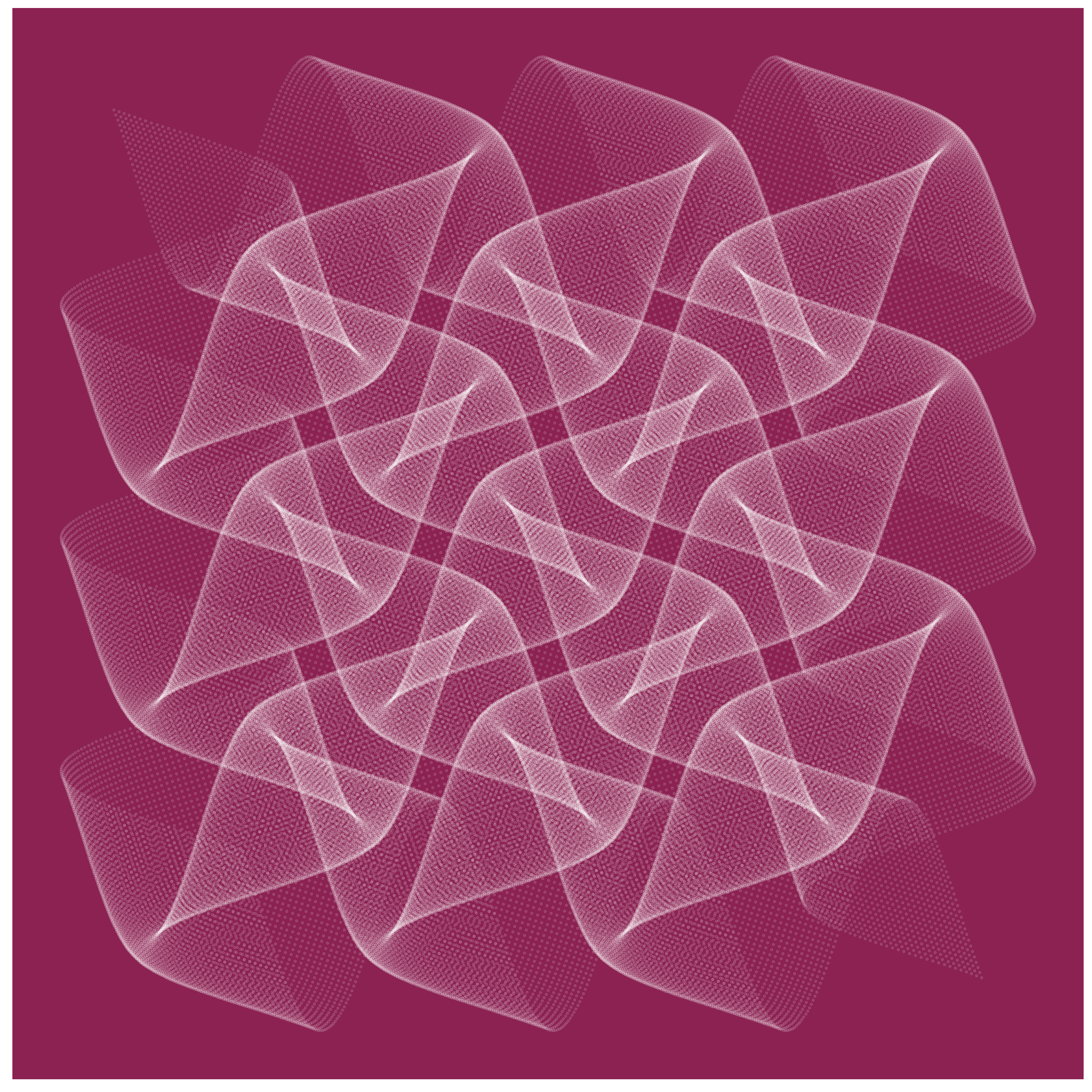# A Silky Drawing and a Tiny Experiment

It is a capital mistake to theorize before one has data (Sherlock Holmes, A Scandal in Bohemia)

One of my favorite entertainments is drawing things: crazy curves, imaginary flowerscelestial bodies, fractalic acacias … but sometimes I wonder myself if these drawings result interesting to whom arrive to my blog. One way to define interesting could be wanting to reproduce the drawing. I know some people do it because they sometimes share with me their creations. So, how many people appreciate the code I write? I manage some a priori for this number (which I will maintain for myself) but I want to refine my estimation with the next experiment. I have done this drawing, which shows that simple mathematics can produce very nice patterns:To estimate how many people is really interested in this plot, at the end of the post I will publish all the code except for a line. If you want the line, you will have to ask it to me. How? It is very easy: you will have to send me a direct message in Twitter. If you don’t follow me, do it here and I will follow you back. If you already follow me but I don’t, tweet something mentioning me and I will follow you back. Then you will be able to send me the direct message. If you prefer, you can send me an email. You can find my email address here.

I know this experiment can be quite biased, but I am also pretty sure that the resulting estimation will be much better than the one I manage nowadays. This is the kidnapped code:

```library(magrittr)
library(ggplot2)
opt = theme(legend.position  = "none",
panel.background = element_rect(fill="violetred4"),
axis.ticks       = element_blank(),
panel.grid       = element_blank(),
axis.title       = element_blank(),
axis.text        = element_blank())
seq(from=-10, to=10, by = 0.05) %>%
expand.grid(x=., y=.) %>%
#HERE COMES THE KIDNAPPED LINE
geom_point(alpha=.1, shape=20, size=1, color="white") + opt
```

## 7 thoughts on “A Silky Drawing and a Tiny Experiment”

1.schulzjan says:

I had to use `geom_point(alpha=.1, shape=20, size=1, color=”white”, stroke=0)` (-> added `stroke=0`) to get a similar picture (windows, latest ggplot package from a day ago)

2.Manoj Oak says:

above code gives

Error: Mapping must be created by `aes()` or `aes_()`

3.E.F.Ibrahim says:

Error: Mapping must be created by `aes()` or `aes_()`
When I tried to display the shape,then the above (ERROR) appeared

What shall I do?

4.ritma001 says:

Wonderful demonstration but I can not reproduce it from the provided code though. Although the aes error is solved by making a dataframe (df) from seq and expand grid and defining ggplot(data = df, x=x, y=y) + geom_point(alpha=.1, shape=20, size=1, color=”white”).

Would be cool to have an updated code!

5.Victor Zurkowski says:

Without “magritter”, editing the previous 2 lines,this works :
W = seq(from=-10, to=10, by = 0.05)
D8 = data.frame(expand.grid(x=W, y=W))
ggplot(data=D8, aes(x=(x+3*sin(y)), y=(y+3*cos(x)))) +
geom_point(alpha=.1, shape=20, size=1, color=”white”) + opt

6.Victor Zurkowski says:

Ah, y que tu R-arte es muy bueno.
Gracias por las contribuciones.

1.@aschinchon says:

Muchas gracias Víctor